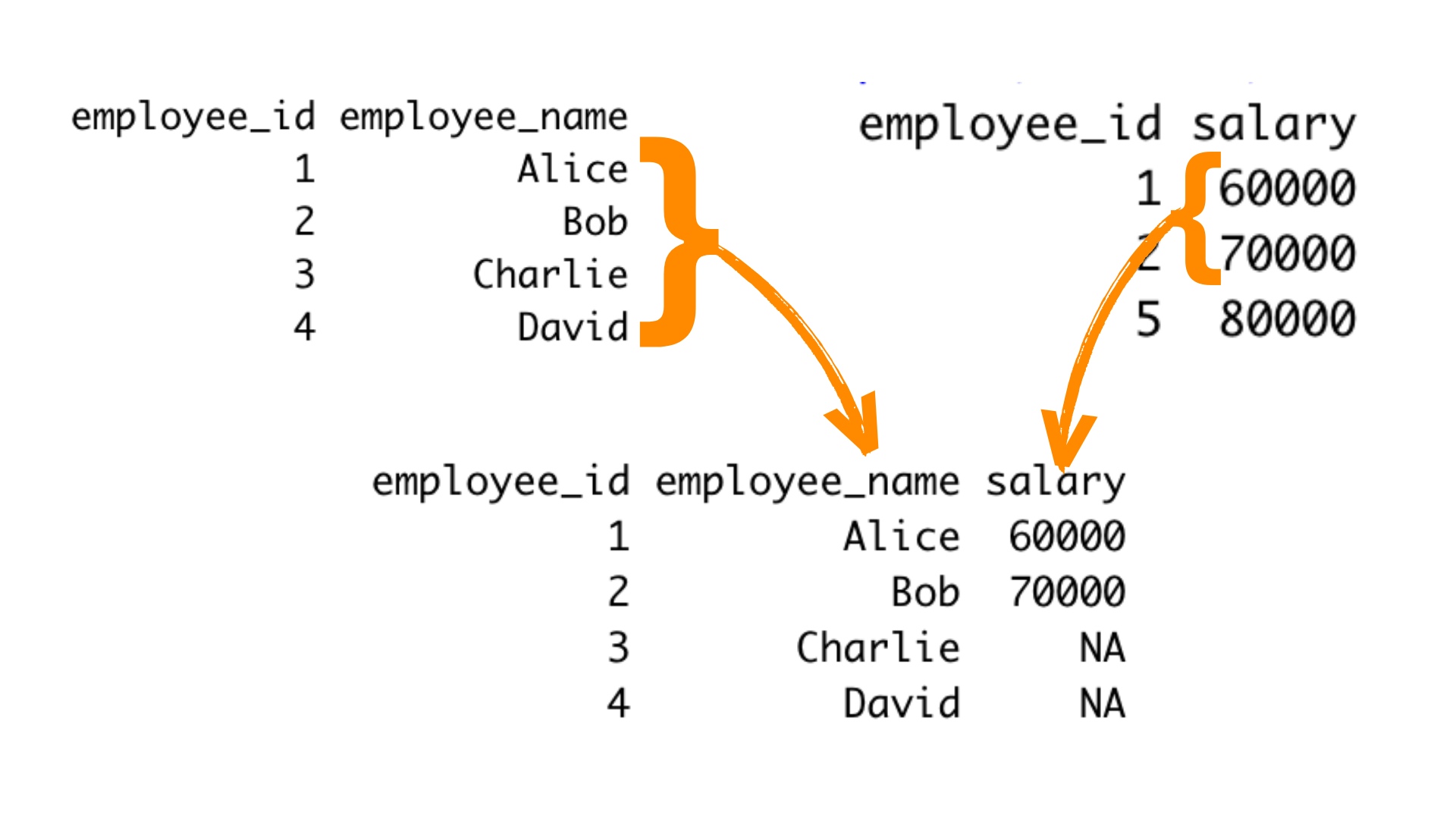# How to do Left Join in R? | 2 Methods (with Examples)

In R, one of the most commonly used tools for data manipulation is the left join. Left joining allows you to combine data from multiple datasets based on a common key column, retaining all rows from the left dataset and matching rows from the right dataset. In this article, we will explore how to perform a left join in R, covering the basics and providing practical examples along the way.

## What is a Left Join in R?

A left join is a type of relational join operation that combines two datasets based on a common column or variable. The result of a left join includes all the rows from the left dataset and any matching rows from the right dataset. If there are no matches in the right dataset, the resulting dataset will still contain all the rows from the left dataset, with missing values (NA) in the columns from the right dataset.

In R, you can perform a left join using the `merge()` function or the dplyr package's `left_join()` function.Let's explore both methods.

### Using the merge() Function

The `merge()` function in R is a versatile tool for combining datasets. To perform a left join, you use the following syntax:

```result <- merge(x, y, by = "common_column", all.x = TRUE)
```

Here:

• `x` is the left dataset.
• `y` is the right dataset.
• `by` specifies the common column(s) to join on.
• `all.x = TRUE` ensures that all rows from the left dataset are retained.

### Using the left_join() Function from dplyr

The dplyr package provides a more intuitive and expressive way to perform data manipulation tasks, including left joins. To use the `left_join()` function, you need to load the dplyr package first. Here's the basic Syntax:

```library(dplyr)

result <- left_join(x, y, by = "common_column")
```

## Practical Examples in R

Let's dive into some practical examples to illustrate how to perform a left join in R.

### Example 1: Left Join with merge()

Suppose you have two datasets: employees and salaries. You want to join these datasets based on the common column "employee_id" to get a complete list of employees with their corresponding salaries.

```employees <- data.frame(
employee_id = c(1, 2, 3, 4),
employee_name = c("Alice", "Bob", "Charlie", "David")
)

salaries <- data.frame(
employee_id = c(1, 2, 5),
salary = c(60000, 70000, 80000)
)

result_merge <- merge(employees, salaries, by = "employee_id", all.x = TRUE)

print(result_merge)
```

In this example, `result_merge` will contain all employees from the `employees` dataset and their corresponding salaries where available. Missing salary information for employees with no matching entry in the `salaries` dataset will be filled with NA.

### Example 2: Left Join with left_join() from dplyr

Let's achieve the same result using the `left_join()` function from the dplyr package.

```library(dplyr)

result_dplyr <- left_join(employees, salaries, by = "employee_id")

print(result_dplyr)
```

The result, stored in `result_dplyr`, will be identical to the result obtained using `merge()`.

### Example 3: Left Join with Multiple Columns

Suppose you have two datasets: `orders` and `customers`. You want to join these datasets based on two common columns, "customer_id" and "order_year," to get a complete list of orders with customer information.

```orders <- data.frame(
order_id = c(1, 2, 3, 4, 5),
customer_id = c(101, 102, 103, 101, 104),
order_year = c(2021, 2022, 2021, 2022, 2022),
product = c("A", "B", "C", "D", "E")
)

customers <- data.frame(
customer_id = c(101, 102, 103, 105),
customer_name = c("Alice", "Bob", "Charlie", "Eve"),
city = c("New York", "Los Angeles", "Chicago", "Houston")
)

result_merge_multi <- merge(orders, customers, by = c("customer_id", "order_year"), all.x = TRUE)

print(result_merge_multi)
```

In this example, `result_merge_multi` will contain all orders from the `orders` dataset with customer information where there are matches based on both "customer_id" and "order_year." Missing customer information for orders with no matching entries in the `customers` dataset will be filled with NA.

The same can be achieved using the `left_join()` function from the dplyr package:

```library(dplyr)

result_dplyr_multi <- left_join(orders, customers, by = c("customer_id", "order_year"))

print(result_dplyr_multi)
```

Performing left joins based on multiple columns allows you to combine data from different sources while considering multiple criteria for matching rows, making it a powerful technique for complex data integration tasks.

## Handling Common Issues

When performing left joins in R, you may encounter common issues. Here are some tips to address them:

1. Duplicate Column Names: If your left and right datasets have columns with the same name but different meanings, consider renaming the columns before the join operation using the `rename()` function from dplyr.
2. Multiple Columns to Join On: If you need to join on multiple columns, you can pass a vector of column names to the by argument. For example, by = c("col1", "col2").

3. Dealing with Missing Values: After the left join, you might encounter missing values (NA) in the result. You can use functions like `na.omit()` or `complete.cases()` to handle or filter out missing data.

4. Check for Data Types: Ensure that the columns you are joining, have compatible data types. If not, you may need to convert them using functions like `as.numeric()` or `as.character()` to change them to numerical and character types respectively.

## Conclusion

Performing a left join in R is a crucial skill for data manipulation and analysis. Whether you choose to use the `merge()` function or the `left_join()` function from the dplyr package, mastering this operation will allow you to combine and analyze data from multiple sources effectively. With the knowledge and examples provided in this article, you are well-equipped to start using left joins in R for your data analysis tasks.

### FavTutor - 24x7 Live Coding Help from Expert Tutors!